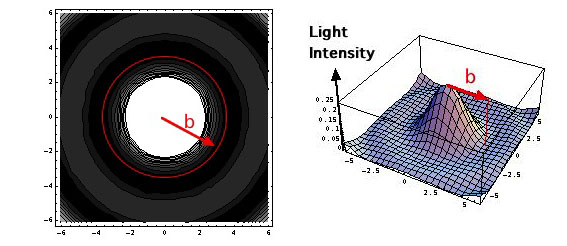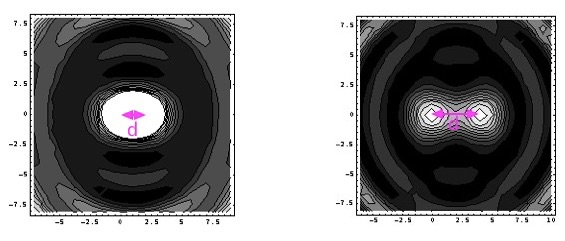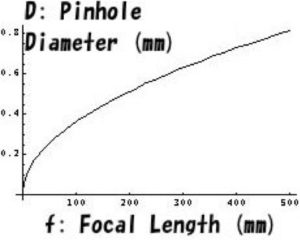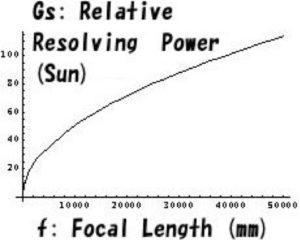# Appendix_5: Resolution of Pinhole Photography

In Appendix_4 we derived the optimum diameter of a pinhole for the given focal length and the given wavelength of the light.  Then how much resolving power is attained by such an optimized “pinhole”?  In other words, how much is the smallest diameter of a sunspot which can be recognized when we observe a sunspot by using the optimized “pinhole lens”?

### Limit of the resolving power

Resolving power is obtained by calculating the distribution of the light intensity of the image of a point source at infinity by a pinhole with a radius of $$a$$.  Though the image of a point source should be a point without area from the mathematical viewpoint, the projected image of the point becomes a circle with a finite diameter $$b$$ as shown below due to the diffraction of the light wave. $$b \cong \frac{3.832}{2\pi} \frac{\lambda f}{a}\cong 0.6098 \frac{\lambda f}{a}$$ where $$\lambda$$ and $$f$$ are the wavelength of light and the distance between the pinhole and a screen.An image of a point source by a pinhole

The left sub-figure shows a contour map of an image of a point source on the focal plane (image screen).  The image becomes a circle with a radius $$b$$ and concentric zones of weak light intensity are seen around the circle.  The right sub-figure shows a 3D schematic figure of the light intensity distribution for the same case.

If images of two point sources keep away by $$d$$and the distance $$d$$ is smaller than the above-mentioned radius $$b (d<b)$$, it is impossible to distinguish these two images (the left sub-figure).  Contrary, for $$d>b$$ two images are distinguishable (the right sub-figure).  Therefore, it is appropriate to consider the distance $$d$$ as the limit of the resolving power.  In a case of a telescope,  $$b/f)$$ is the definition of the resolving power.Images of two source points and the resolution
The left sub-figure: Images of two point sources placed aloof by $$d(<b)$$ are not distinguishable. The right sub-figure: Images of two point sources placed aloof by $$d (>b)$$ are distinguishable.

### Relative Resolving Power

When one would take a photograph of sunspots so that the image of the sun fills up the whole imaging screen, relation between the length of a side $$S$$ of an imaging screen (a sensor) and the resolving power $$b$$ becomes important and we define the relative resolving power $$G(=S/b)$$.  This relative resolving power is rewritten as a function of a wavelength of the light $$\lambda$$, the focal length $$f$$, and the size of the sensor $$S$$, and it is expressed as $$G \cong \frac{1.28S}{\sqrt{\lambda f}}$$ Though in the case of a photograph or a computer display the resolving power is usually expressed as “a number of pixels per unit length”, the relative resolving power defined here is “a number of pixels per a side of a square sensor”.  As astronomical objects such as the sun are located at infinity a size of an object is expressed not by a length but by an angle viewing the object.  As the relation between the viewing angle $$\theta$$ and the size of the object is expressed as $$S=\theta f$$,  the relative resolving power is derived as $$G \cong 1.28 \theta \sqrt{\frac{f}{\lambda}}$$  This means that the relative resolving power is increased by increasing the focal length and the magnification factor.  If the apparent diameter of the sun, about 32’(=0.00931 radian), as adopted as the viewing angle, the relative resolving power for the sun is $$G_{sun}\cong 0.51 \sqrt{f}$$ where the unit of $$f$$ is millimeter.  It should be noted that the equation is derived for the case of constant viewing angle $$\theta$$.  Therefore, the size of the imaging screen becomes larger with increasing focal length.  When one takes a photograph by loading the “pinhole lens” to a ready-made camera, such as a SLR, the formula of the relative resolving power should be a function of $$S$$, $$f$$ and $$\lambda$$ as $$G \cong \frac{1.28S}{\sqrt{\lambda f}}$$.   This equation means that the relative resolving power is improved by increasing the focal length $$f$$ to increase the multiplication factor.  As the apparent diameter of the sun is about $$32’$$(=0.00931 radian), for the wavelength of $$550 nm$$ this equation is reduced to  $$G_{sun}\cong 0.51 \sqrt{f}$$ It should be noted that this equation can be used for the constant value of viewing angle $$\theta$$, which is the case the size of the imaging screen is increased with increasing focal length.  Therefore, for taking a photograph by using a camera with a pinhole it is necessary to use the equation $$G \cong \frac{1.28S}{\sqrt{\lambda f}}$$  It should be remarked that the resolving powers of these cases depend on $$f$$ inversely.

### Summary

For reference we show some typical graphs: a pinhole diameter as a function of the focal length, $$D \cong 0.0366 \sqrt{f}$$, the relative resolving power for observation of the sun, $$G_{sun} \cong 0.51 \sqrt{f}$$, and the resolving power as a function of the size of the imaging plane $$S$$, $$G \cong 55S/\sqrt{f}$$, where the wavelength of the incident light is $$550 nm$$.Pinhole diameter vs. focal lengthPinhole diameter versus focal lengthRelative resolving power versus focal length for a fixed viewing angle (the Sun)Resolving power versus focal length for a fixed size of a picture screen